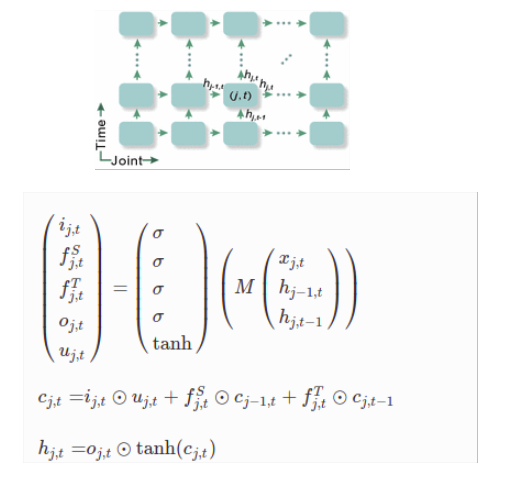# Why is my LSTM model not learning?

Hi, I’m trying to implement spatio-temporal LSTM (ST-LSTM) model for human action recognition using 3D skeleton data, basis on this article: Spatio-Temporal LSTM with Trust Gates for 3D Human Action Recognition | SpringerLink.

Neural network model and single ST-LSTM equations looks like below:as input to ST-LSTM I pass hidden and cell state from previous ST-LSTM in temporal and spatial dimension together with single joint (x, y, z) as input. Shapes of input vectors are:

torch.Size([BATCH_SIZE, 128]) - hidden state
torch.Size([BATCH_SIZE, 128]) - cell state
torch.Size([BATCH_SIZE, 3]) - input (x, y, z) coordinate of single joint

I implemented ST-LSTM cell as below:

``````STLSTMState = namedtuple('STLSTMState', ['h_temp_prev', 'h_spat_prev', 'c_temp_prev', 'c_spat_prev'])

class STLSTMCell(RNNCellBase):
def __init__(self, input_size: int, hidden_size: int, bias: bool = True) -> None:
super(STLSTMCell, self).__init__(input_size, hidden_size, bias, num_chunks=5)
self.input_size = input_size
self.hidden_size = hidden_size
self.w_ih = Parameter(torch.randn(5 * hidden_size, input_size))
self.w_hh0 = Parameter(torch.randn(5 * hidden_size, hidden_size))
self.w_hh1 = Parameter(torch.randn(5 * hidden_size, hidden_size))
self.b_ih = Parameter(torch.randn(5 * hidden_size))
self.b_hh0 = Parameter(torch.randn(5 * hidden_size))
self.b_hh1 = Parameter(torch.randn(5 * hidden_size))

def forward(self, input: Tensor, state: Optional[Tuple[Tensor, Tensor, Tensor, Tensor]]) -> Tuple[Tensor, Tensor]:
self.check_forward_input(input)
self.check_forward_hidden(input, state, '')
self.check_forward_hidden(input, state, '')
self.check_forward_hidden(input, state, '')
self.check_forward_hidden(input, state, '')
return self.lstm_cell(
input, state,
self.w_ih, self.w_hh0, self.w_hh1,
self.b_ih, self.b_hh0, self.b_hh1,
)

def lstm_cell(self, input, state, w_ih, w_hh0, w_hh1, b_ih, b_hh0, b_hh1):
# type: (Tensor, Tuple[Tensor, Tensor, Tensor, Tensor], Tensor, Tensor, Tensor, Tensor) -> Tuple[Tensor, Tensor]
h_temp_prev, h_spat_prev, c_temp_prev, c_spat_prev = state
gates = (torch.mm(input, w_ih.t())
+ torch.mm(h_spat_prev, w_hh0.t())
+ torch.mm(h_temp_prev, w_hh1.t()))
gates += b_ih + b_hh0 + b_hh1

in_gate, forget_gate_s, forget_gate_t, out_gate, u_gate = gates.chunk(5, 1)

in_gate = torch.sigmoid(in_gate)
forget_gate_s = torch.sigmoid(forget_gate_s)
forget_gate_t = torch.sigmoid(forget_gate_t)
out_gate = torch.sigmoid(out_gate)
u_gate = torch.tanh(u_gate)

cy = (in_gate * u_gate) + (forget_gate_s * c_spat_prev) + (forget_gate_t * c_temp_prev)
hy = out_gate * torch.tanh(cy)

return hy, cy
``````

my model:

``````class STLSTMModel(nn.Module):
def __init__(self, input_size, hidden_size, batch_size, dropout, classes_count):
super(STLSTMModel, self).__init__()
self.input_size = input_size
self.hidden_size = hidden_size
self.batch_size = batch_size
self.st_lstm_cell_1 = STLSTMCell(input_size, hidden_size)
self.st_lstm_cell_2 = STLSTMCell(hidden_size, hidden_size)
self.fc = torch.nn.Linear(hidden_size, classes_count)
self.dropout_l = nn.Dropout(dropout)

def forward(self, input, state):
h_next, c_next = self.st_lstm_cell_1(input, state)
h_next = self.dropout_l(h_next)
out, c_next = self.st_lstm_cell_2(h_next, state)
out = self.fc(out)
return h_next, c_next, F.log_softmax(out, dim=-1)
``````

my training loop:

``````learning_rate = 0.002
momentum = 0.9
weight_decay = 0.95
dropout = 0.5

st_lstm_model = STLSTMModel(input_size, hidden_size, batch_size, dropout, len(classes)).to(device)

criterion = nn.NLLLoss()
optimizer = optim.SGD(st_lstm_model.parameters(), lr=learning_rate, momentum=momentum, weight_decay=weight_decay)

all_losses = []

for epoch in range(epoch_nb):
data, train_y = get_data()
tensor_train_y = torch.from_numpy(np.array(train_y)).to(device)

joints_count = data.shape
spatial_dim = joints_count
temporal_dim = data.shape

cell1_out = [[[None, None] for _ in range(spatial_dim)] for _ in range(temporal_dim)]

losses_arr = []

for t in range(temporal_dim):
for j in range(spatial_dim):
if j == 0:
h_spat_prev = torch.zeros(batch_size, hidden_size).to(device)
c_spat_prev = torch.zeros(batch_size, hidden_size).to(device)
else:
h_spat_prev = cell1_out[t][j - 1]
c_spat_prev = cell1_out[t][j - 1]
if t == 0:
h_temp_prev = torch.zeros(batch_size, hidden_size).to(device)
c_temp_prev = torch.zeros(batch_size, hidden_size).to(device)
else:
h_temp_prev = cell1_out[t - 1][j]
c_temp_prev = cell1_out[t - 1][j]
state = STLSTMState(h_temp_prev, h_spat_prev, c_temp_prev, c_spat_prev)
input = data[t][j]
h_next, c_next, output = st_lstm_model(torch.tensor(input, dtype=torch.float, device=device), state)
cell1_out[t][j] = h_next
cell1_out[t][j] = c_next
losses_arr.append(criterion(output, tensor_train_y))

loss = 0
for l in losses_arr:
loss += l
loss /= (spatial_dim * temporal_dim)

loss.backward()
optimizer.step()
``````

I implemented this exactly as it’s described in article, but my training loss is not failing even after 5K iterations (it is around 2.4).

I tried also to use this same data and instead ST-LSTM use my custom implementation of LSTMCell where as input I passed Tensor with the entire action sequence (20 frames) containing all keypoints (12 kpts * (x,y,z) → 36 inputs)

torch.Size([5, 128]) - hidden state
torch.Size([5, 128]) - cell state
torch.Size([5, 20, 36]) - input

and it works like a charm - loss function is around 0.1 after 1K iterations and after 5K it’s around 0.0001

Do You know why my ST-LSTM model is not working? I spend several days trying to debug it and I have no idea what can be wrong.